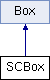ParM  parm A molecular dynamics library
SCBox Class Reference

A spheocylinder box, also known as a capsule. More...

#include <box.hpp>

Inheritance diagram for SCBox:## Public Member Functions

SCBox (flt L, flt R)

Vec diff (Vec r1, Vec r2)
Distance between two points, given boundary conditions. More...

flt V ()
Volume. Can return NaN. More...

Vec dist (Vec r1)

Vec edge_dist (Vec r1)

bool inside (Vec r1, flt buffer=0.0)

Vec rand_loc (flt min_dist_to_wall=0.0)

flt length ()Public Member Functions inherited from Box
virtual ~Box ()

flt L

flt R

## Detailed Description

A spheocylinder box, also known as a capsule.

Note that this does not keep particles inside the box; use an Interaction like SCBoxed for that.

This class is useful for functions like V(), dist, edge_dist, inside, rand_loc, etc.

The spherocylinder has an axis along the x-axis, centered at origin

L is length of the central axis of the cylinder from 1 sphere center to the other, so L=0 is a sphere.

## Constructor & Destructor Documentation

 SCBox::SCBox ( flt L, flt R )

## Member Function Documentation

 Vec SCBox::diff ( Vec r1, Vec r2 )
inlinevirtual

Distance between two points, given boundary conditions.

This is the main function that Box exists for.

Implements Box.

 Vec SCBox::dist ( Vec r1 )
 Vec SCBox::edge_dist ( Vec r1 )
 bool SCBox::inside ( Vec r1, flt buffer = 0.0 )
 flt SCBox::length ( )
inline
 flt SCBox::radius ( )
inline
 Vec SCBox::rand_loc ( flt min_dist_to_wall = 0.0 )
 flt SCBox::V ( )
virtual

Volume. Can return NaN.

Implements Box.

## Member Data Documentation

 flt SCBox::L
protected
 flt SCBox::R
protected

The documentation for this class was generated from the following files: